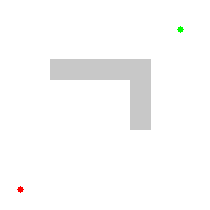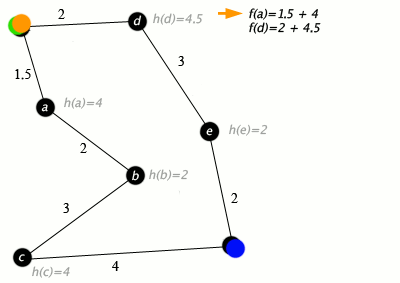# How does the A* Algorithm work and What is it?

views:0

Path Finding has been one of the oldest and most popular applications in computer programming. You could virtually find the most optimal path from a source to a destination by adding costs which would represent time, money etc. A* is one of the most popular algorithms for all the right reasons. In this article, let’s find out just why.

And if you are looking to get certified and Artificial Intelligence and Machine Learning , join the various programs offered by Mildaintrainings today!

Let’s get started ?

### What is a Search Algorithm?

Motivation behind a Path Search Algorithm is To approximate the shortest path in real-life situations, like- in maps, games where there can be many hindrances. Moving from one place to another is a task that we humans do almost every day. We try to find the shortest path possible that enables us to reach our destinations faster and make the whole process of travelling as efficient as possible.

We have algorithms that can help us find the shortest paths virtually. We just need to add costs (time, money etc.) to the graphs or maps and the algorithm finds us the path that we need to take to reach our destination as quick as possible. Many algorithms were developed through the years for this problem and A* is one the most popular algorithms out there.

### What is A* Search Algorithm? ?

A* (pronounced “A-star”) is a graph traversal and path search algorithm, which is often used in many fields of computer science due to its completeness, optimality, and optimal efficiency. A* Search algorithm is one of the best and popular technique used in path-finding and graph traversals.

### Why A* Search Algorithm ?

Informally speaking, A* Search algorithms, unlike other traversal techniques, it has “brains”. What it means is that it is really a smart algorithm which separates it from the other conventional algorithms. And it is also worth mentioning that many games and web-based maps use this algorithm to find the shortest path very efficiently (approximation).

A* is an advanced BFS algorithm that searches for shorter paths first rather than the longer paths. A* is optimal as well as a complete algorithm.

What do I mean by Optimal and Complete? Optimal meaning that A* is sure to find the least cost from the source to the destination and Complete meaning that it is going to find all the paths that are available to us from the source to the destination.

So that makes A* the best algorithm right? Well, in most cases, yes. But A* is slow and also the space it requires is a lot as it saves all the possible paths that are available to us. This makes other faster algorithms have an upper hand over A* but it is nevertheless, one of the best algorithms out there.

### So why choose A* over other faster algorithms?

Let the graphs below answer that for you. I have taken the Dijkstra’s algorithm and A* Algorithm for comparison.

You can see here that the Dijkstra’s Algorithm finds all the paths that can be taken without finding or knowing which is the most optimal one for the problem that we are facing. This leads to the unoptimized working of the algorithm and unnecessary computations.Dijkstra’s Algorithm (Wikipedia)

A* algorithm, on the other hand, finds the most optimal path that it can take from the source in reaching the destination. It knows which is the best path that can be taken from its current state and how it needs to reach its destination.A* Algorithm (Wikipedia)

### The in-and-out of A* Algorithm

Now that you know why we choose A*, let’s understand a bit of theory about it as it is essential to help you understand how this algorithm works.

A*, as we all know by now, is used to find the most optimal path from a source to a destination. It optimizes the path by calculating the least distance from one node to the other.

There is one formula that all of you need to remember as it is the heart and soul of the algorithm.

### "f = g + h"

Explanation :

Consider a square grid having many obstacles and we are given a starting cell and a target cell. We want to reach the target cell (if possible) from the starting cell as quickly as possible. Here A* Search Algorithm comes to the rescue.

What A* Search Algorithm does is that at each step it picks the node according to a value- ‘f’ which is a parameter equal to the sum of two other parameters – ‘g’ and ‘h’ . At each step it picks the node/cell having the lowest ‘f’ , and process that node/cell.

We define ‘g’ and ‘h’ as simply as possible below

g = the movement cost to move from the starting node/point to a given square/node on the grid, following the path generated to get there.

h = the estimated movement cost to move from that given node/square on the grid to the final destination. This is often referred to as the heuristic, which is nothing but a kind of smart guess. We really don’t know the actual distance until we find the path, because all sorts of things can be in the way (walls, water, etc.). There can be many ways to calculate this ‘h’ .

So once that you have understood this formula, let me just show you a simple example to help you understand how this algorithm works.A* Algorithm (Wikipedia)

Key : green: start; blue: goal; orange: visited
Algorithm
We create two lists – Open List and Closed List (just like Dijkstra Algorithm)

// A* Search Algorithm

1. Initialize the open list
2. Initialize the closed list
put the starting node on the open
list (you can leave its f at zero)

3. while the open list is not empty

i) if successor is the goal, stop search
successor.g = q.g + distance between
successor and q
successor.h = distance from goal to
successor (This can be done using many
ways, we will discuss three heuristics-
Manhattan, Diagonal and Eucl
idean
Heuristics)

successor.f = successor.g + successor.h

ii) if a node with the same position as
successor is in the OPEN list which has a
lower f than successor, skip this successor

iii) if a node with the same position as
successor is in the CLOSED list which has
a lower f than successor, skip this successor
otherwise, add the node to the open list
end (for loop)

e) push q on the closed list
end (while loop)

Limitations

Although being the best pathfinding algorithm around, A* Search Algorithm doesn’t produce the shortest path always, as it relies heavily on heuristics / approximations to calculate – h

Applications

This is the most interesting part of A* Search Algorithm. They are used in games! But how?

Ever played Tower Defense Games ?

Tower defense is a type of strategy video game where the goal is to defend a player’s territories or possessions by obstructing enemy attackers, usually achieved by placing defensive structures on or along their path of attack.

A* Search Algorithm is often used to find the shortest path from one point to another point. You can use this for each enemy to find a path to the goal.

One example of this is the very popular game- Warcraft II

### Source Code (in Python ?)

class Node() : “””A node class for A* Pathfinding”””
def __init__ (self, parent=None, position=None):
self.parent = parent
self.position = position
self.g = 0
self.h = 0
self.f = 0
def __eq__ (self, other):
return self.position == other.position
defastar (maze, start, end):
“””Returns a list of tuples as a path from the given start to the given end in the given maze”””
# Create start and end node
start_node = Node(None, start)
start_node.g = start_node.h = start_node.f = 0
end_node =Node (None, end)
end_node.g = end_node.h = end_node.f = 0
# Initialize both open and closed list
open_list = []
closed_list = []
open_list. append(start_node)
# Loop until you find the end
whilelen (open_list) > 0:
# Get the current node
current_node = open_list
current_index = 0
for index, item in enumerate (open_list):
if item.f < current_node.f:
current_node = item
current_index = index
# Pop current off open list, add to closed list
open_list.pop(current_index)
closed_list.append(current_node)
# Found the goal
if current_node == end_node:
path = []
current = current_node
while current is not None:
path.append(current.position)
current = current.parent
return path[::–1] # Return reversed path
# Generate children
children = []
for new_position in [(0, –1), (0, 1), (–1, 0), (1, 0), (–1, –1), (–1, 1), (1, –1), (1, 1)]: # Adjacent squares # Get node position
node_position = (current_node.position + new_position, current_node.position + new_position)
# Make sure within range
if node_position > (len(maze) – 1) or node_position < 0 or node_position > (len(maze[len(maze)–1]) –1) or node_position < 0:
continue
# Make sure walkable terrain
if maze[node_position][node_position] != 0:
continue
# Create new node
new_node = Node(current_node, node_position)
# Append
children.append(new_node)
# Loop through children
for child in children:
# Child is on the closed list
for closed_child in closed_list:
if child == closed_child:
continue
# Create the f, g, and h values
child.g = current_node.g + 1
child.h = ((child.position – end_node.position) ** 2) + ((child.position – end_node.position) ** 2) child.f = child.g + child.h

# Child is already in the open list
for open_node in open_list:
if child == open_node and child.g > open_node.g:
continue
# Add the child to the open list
open_list.append(child)
def main():
maze = [[0, 0, 0, 0, 1, 0, 0, 0, 0, 0],
[0, 0, 0, 0, 1, 0, 0, 0, 0, 0],
[0, 0, 0, 0, 1, 0, 0, 0, 0, 0],
[0, 0, 0, 0, 1, 0, 0, 0, 0, 0],
[0, 0, 0, 0, 1, 0, 0, 0, 0, 0],
[0, 0, 0, 0, 0, 0, 0, 0, 0, 0],
[0, 0, 0, 0, 1, 0, 0, 0, 0, 0],
[0, 0, 0, 0, 1, 0, 0, 0, 0, 0],
[0, 0, 0, 0, 1, 0, 0, 0, 0, 0],
[0, 0, 0, 0, 0, 0, 0, 0, 0, 0]]
start = (0, 0)
end = (7, 6)
path = astar(maze, start, end)
print(path)
if __name__ == ‘__main__’:
main()
view raw

View More >>

Now that you know about the A* Algorithm, check out our Artificial Intelligence Course offered by Mildaintrainings , a trusted online learning company with a network of more than 115,000 learners spread across the globe.

Artificial Intelligence is the simulation of human intelligence through machines & mostly through computer systems. It allows computers to do things which are normally done by human beings. Any program can be said to be Artificial Intelligence if it is able to do something that the humans do it using their intelligence through AI. AI is a broad topic ranging from simple calculators to self-steering technology to something that might radically change the future. Learn Artificial Intelligence course by the industry experts, the program is conducted by Mildaintrainings

Artificial Intelligence for better tomorrow. Enroll & Get Certified now!

## Drop us a Query// load third party scripts onload